# Change Nickname

Current Nickname:
Search
v4.0
v4.0

# HANP

## Overview

HANP (Hop Attenuation & Node Preference) algorithm is an extension of the Label Propagation algorithm (LPA). It introduces a label score attenuation mechanism based on LPA, and considers the influence of neighbor node degree on neighbor label weight. The algorithm was proposed by Ian X.Y. Leung in 2009.

Related material of the algorithm:

## Basic Concept

### Label Score

Label score indicates the propagation ability of the label. The HANP algorithm assigns all labels initial score of 1, and each time a node adopts new label from its neighbor (i.e. the label hops once), the score of the new label being propagated to that node will have a hop attenuation, this is what HA (Hop Attenuation) means in the name of the algorithm. The hop attenuation is denoted as `δ`, which can prevent large cluster from dominating. In the graph below, given `δ = 0.3`, when the green node adopts label `a`, the score of label `a` on the green node would attenuate to `2 - 0.3 = 1.7`.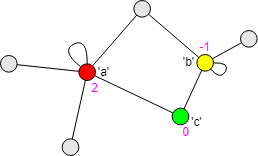When the adopted new label comes from multiple neighbors, the score of the label is attenuated from the maximum score.

### Preference For Node Degree

Preference for node degree means that node with greater degree has better ability to propagate labels; in other words, a node tends to adopt label from neighbor with greater degree, this is what NP (Node Preference) means in the name of the algorithm.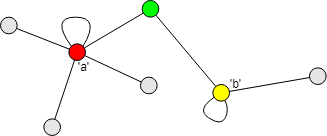In the graph above, the green node has two neighbors - the red node with degree of 6 (self-loop edge is counted twice), and the yellow node with the degree of 4, so the green node prefers to adopt label `a`.

Of course, it is also possible to prioritize the propagation of label of node with lower degrees. Therefore, the algorithm considers the exponential power of the degree of the node where the label is located. When the power exponent is greater than 0, labels with high node degree will be propagated preferentially; when the power exponent is less than 0, labels with low node degree are preferentially propagated; when the power exponent is 0, the node degree does not affect the priority of label propagation.

### Label Weight

Node `j` has label `l`, when it propagates to its neighbor node `i`, the weight of label `l` (i.e. `w(l)`) equals to the product of the score of `l` on `j` (i.e. `s(j)`), the exponential power function of the degree of `j`, and the weight of all edges between `i` and `j`; when `i` has multiple neighbor nodes with label `l`, the weight of each `l` needs to be summed: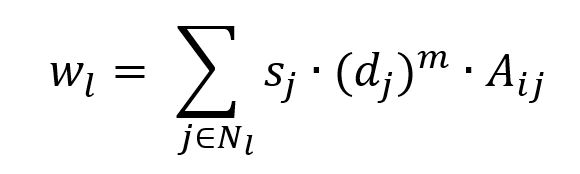where `d(j)` is the degree of `j`, `m` is the power exponent, `A(ij)` is the total weight of all edges between `i` and `j`.

For any node `i` in the graph, label `l` is on `i`'s neighbor, then `i`'s new label `l'` can be denoted as: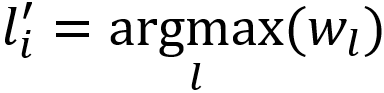Let `s(l')` be the score of the label on the neighbor nodes, `δ` is the hop attenuation, so the score of the new label `l'` on `i` is: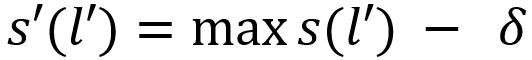Please note, `δ = 0` if the selected label is equal to the current label, i.e. the score of the label does not change.

The iteration process of the HANP algorithm is similar to LPA. All label scores are set to 1 at the beginning; during each iteration, it calculates for each node whether there is any label different from its current label or any label same with its current label but has higher score that can be adopted from its neighbors; and after all nodes are calculated, updates the nodes which can be updated. Iterating and looping by this rule until no node could adopt new label or update label score, or the number of iterations reaches the limit.

Different from LPA, since the HANP algorithm applies label score, which can prevent label oscillations, no extra interrupt mechanism is needed.

## Special Case

### Lonely Node, Disconnected Graph

For disconnected graph, there is no edge between its lonely nodes and connected components to propagate labels, connected components do not contain the same initial labels will not be divided into the same community.

### Self-loop Edge

The HANP algorithm treats the self-loop edge in the same way with the Degree algorithm `algo(degree)`, which counts each self-loop edge twice.

### Directed Edge

For directed edges, the HANP algorithm ignores the direction of edges but calculates them as undirected edges.

## Results and Statistics

Run HANP algorithm in the graph below, all node and edge weights are 1, the letter wrote in the node is the initial label of node, node 16 has no label, the algorithm iterates 10 rounds, the power exponent `m` is 0.1, hop attenuation `δ` is 0.2: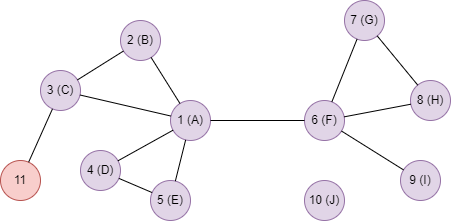Algorithm results: Keep maximum one label for each node, return `_uuid`, `label_1` and `score_1`

_uuid label_1 score_1
1 A -0.200000
2 F -0.200000
3 F -0.200000
4 F -0.200000
5 F -0.200000
6 A -0.200000
7 A -0.200000
8 A -0.200000
9 A -0.200000
10 J 1
11

Algorithm statistics: Number of labels `label_count`

label_count
3

## Command and Configuration

• Command: `algo(hanp)`
• Configurations for the parameter `params()`:
Name
Type
Default
Specification Description
loop_num int 5 >=1 Number of iterations
node_label_property `@<schema>.<property>` / Numeric or string class node property, LTE needed Name of the node schema and property as label; node without this property will not participate in the calculation; random number is used as label if not set
edge_weight_property `@<schema>.<property>` / Numeric edge property, LTE needed Name of the edge schema and property as edge weight; edge without this property will not participate in the calculation; weight is 1 if not set
m float / Mandatory The power exponent of the degree of neighbor node, which indicates the preference of node degree; `m = 0` means to ignore node degree, `m > 0` means to prioritize neighbors with high degree, `m < 0` means to prioritize neighbors with low degree
delta float / (0,1); mandatory Hop attenuation of label score during propagation
limit int -1 >= -1 Number of results to return; return all results if sets to -1 or not set

Example: Run HANP, label locates on node property @default.label, edge weight locates on edge property @default.level, the power exponent is 0.1, hop attenuation is 0.2, iterate 10 rounds

``````algo(hanp).params({
loop_num: 10,
node_label_property: "@default.label",
edge_weight_property: "@default.level",
m: 0.1,
delta: 0.2
}) as a return a
``````

## Algorithm Execution

#### 1. File Writeback

Configuration
Data in Each Row
filename `_id`,`label_1`,`score_1`

Example: Run HANP, label locates on node property @default.label, edge weight locates on edge property @default.level, the power exponent is 0.1, hop attenuation is 0.2, iterate 10 rounds, write the algorithm results back to file named hanp

``````algo(hanp).params({
loop_num: 10,
node_label_property: "@default.label",
edge_weight_property: "@default.level",
m: 0.1,
delta: 0.2
}).write({
file:{
filename: "hanp"
}
})
``````

#### 2. Property Writeback

Configuration
Writeback Content
Type
Data Type
property `label_1`,`score_1` Node property Data type of label is `string`, data type of label score is `float`

Example: Run HANP, label locates on node property @default.label, weight of each edge is 1, the power exponent is 0.1, hop attenuation is 0.2, iterate 10 rounds, write the algorithm results back to node properties named tag_1 and score_1

``````algo(hanp).params({
loop_num: 10,
node_label_property: "@default.label",
m: 0.1,
delta: 0.2
}).write({
db:{
property: "tag"
}
})
``````

#### 3. Statistics Writeback

Name Data Type Description
`label_count` int Number of labels

The number of labels means the number of labels left after the algorithm is finished; the HANP algorithm only keeps one label the maximum for each node, the number of labels is the number of communities.

Example: Run HANP, label locates on node property @default.label, weight of each edge is 1, the power exponent is 0.1, hop attenuation is 0.2, iterate 10 rounds, write the algorithm statistics back to task information

``````algo(hanp).params({
loop_num: 10,
node_label_property: "@default.label",
m: 0.1,
delta: 0.2
}).write()
``````

### Direct Return

Alias Ordinal
Type
Description Column Name
0 []perNode Node and its label, label score `_uuid`, `label_1`, `score_1`
1 KV Number of labels `label_count`

Example: Run HANP, label locates on node property @default.label, weight of each edge is 1, the power exponent is 0.1, hop attenuation is 0.2, iterate 10 rounds, define algorithm results and statistics as aliases named results and count, and return the results and statistics

``````algo(hanp).params({
loop_num: 10,
node_label_property: "@default.label",
m: 0.1,
delta: 0.2
}) as results, count
return results, count
``````

### Streaming Return

Alias Ordinal
Type
Description Column Name
0 []perNode Node and its label, label score `_uuid`, `label_1`, `score_1`

Example: Run HANP, label locates on node property @default.label, weight of each edge is 1, the power exponent is 0.1, hop attenuation is 0.2, iterate 10 rounds, and return the results ordered by the ascending label name and node UUID

``````algo(hanp).params({
loop_num: 10,
node_label_property: "@default.label",
m: 0.1,
delta: 0.2
}).stream() as res
return res order by res.label_1, res._uuid
``````

### Real-time Statistics

Alias Ordinal
Type
Description Column Name
0 KV Number of labels `label_count`

Example: Run HANP, label locates on node property @default.label, weight of each edge is 1, the power exponent is 0.1, hop attenuation is 0.2, iterate 10 rounds, and return the number of communities

``````algo(hanp).params({
loop_num: 10,
node_label_property: "@default.label",
m: 0.1,
delta: 0.2
}).stats() as count
return count
``````

## Consistency of Results

Affected by factors such as the order of nodes, the random selection of labels with the same weights, the parallel calculation and so on, the community division results of the HANP algorithm may be different.